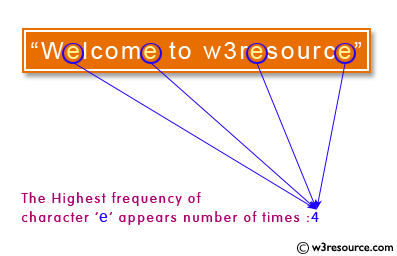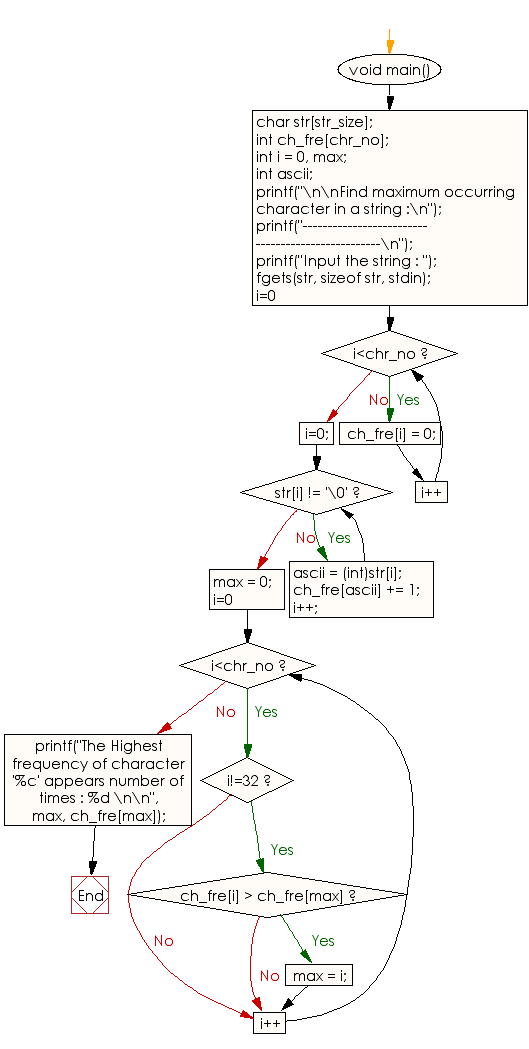﻿ C exercises: Find maximum occurring character in a string - w3resource# C Programming: Find maximum occurring character in a string

## C String: Exercise-10 with Solution

Write a program in C to find maximum occurring character in a string.Sample Solution:

C Code:

``````#include <stdio.h>
#include <string.h>
#include <stdlib.h>

#define str_size 100 //Declare the maximum size of the string
#define chr_no 255 //Maximum number of characters to be allowed

void main()
{
char str[str_size];
int ch_fre[chr_no];
int i = 0, max;
int ascii;

printf("\n\nFind maximum occurring character in a string :\n");
printf("--------------------------------------------------\n");
printf("Input the string : ");
fgets(str, sizeof str, stdin);

for(i=0; i<chr_no; i++)  //Set frequency of all characters to 0
{
ch_fre[i] = 0;
}

/* Read for frequency of each characters */
i=0;
while(str[i] != '\0')
{
ascii = (int)str[i];
ch_fre[ascii] += 1;

i++;
}

max = 0;
for(i=0; i<chr_no; i++)
{
if(i!=32)
{
if(ch_fre[i] > ch_fre[max])
max = i;
}
}
printf("The Highest frequency of character '%c' appears number of times : %d \n\n", max, ch_fre[max]);
}
```
```

Sample Output:

```Find maximum occurring character in a string :
--------------------------------------------------
Input the string : Welcome to w3resource
The Highest frequency of character 'e' appears number of times : 4
```

Flowchart:C Programming Code Editor:

Improve this sample solution and post your code through Disqus.

What is the difficulty level of this exercise?

﻿